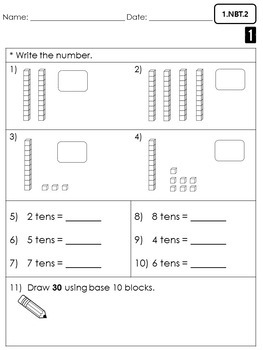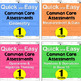# 1st Grade Common Core Math Assessments- FREEBIESubject
Resource Type
File Type

PDF

(3 MB)
Standards
• Product Description
• StandardsNEW

This is a sample packet of the big bundle of 1st grade common core math assessments. Your feedback will be very appreciated :) Thank you!

It includes one assessment from each 1st grade common core math standard:

1. Operations & Algebraic Thinking (OA)

2. Number & Operations in Base Ten (NBT)

3. Measurement & Data (MD)

4. Geometry (G)

You can purchase a huge bundle of all 4 packets here:

First Grade Math Assessments COMPLETE PACKET

You can click these links to look at the individual packets:

First Grade Math Assessments- OA Standards

First Grade Math Assessments- NBT Standards

First Grade Math Assessments- MD Standards

First Grade Math Assessments- G Standards

Other materials you may be interested:

1st Grade Common Core Language Practice Sheets : CONVENTIONS AND GRAMMAR

1st Grade Common Core Language Practice Sheets : VOCABULARY ACQUISITION

Easy Print Phonics Printables FREEBIE

Thank you for visiting my little store! :D

Tiny Teaching Shack

Partition circles and rectangles into two and four equal shares, describe the shares using the words halves, fourths, and quarters, and use the phrases half of, fourth of, and quarter of. Describe the whole as two of, or four of the shares. Understand for these examples that decomposing into more equal shares creates smaller shares.
Compose two-dimensional shapes (rectangles, squares, trapezoids, triangles, half-circles, and quarter-circles) or three-dimensional shapes (cubes, right rectangular prisms, right circular cones, and right circular cylinders) to create a composite shape, and compose new shapes from the composite shape.
Distinguish between defining attributes (e.g., triangles are closed and three-sided) versus non-defining attributes (e.g., color, orientation, overall size); build and draw shapes to possess defining attributes.
Determine the unknown whole number in an addition or subtraction equation relating three whole numbers. For example, determine the unknown number that makes the equation true in each of the equations 8 + ? = 11, 5 = ▯ - 3, 6 + 6 = ▯.
Understand the meaning of the equal sign, and determine if equations involving addition and subtraction are true or false. For example, which of the following equations are true and which are false? 6 = 6, 7 = 8 - 1, 5 + 2 = 2 + 5, 4 + 1 = 5 + 2.
Total Pages
N/A
N/A
Teaching Duration
N/A
Report this Resource to TpT
Reported resources will be reviewed by our team. Report this resource to let us know if this resource violates TpT’s content guidelines.